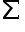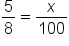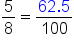+

Author: Sophia Tutorial
##### Description:

Examine ways to represent variables in a tabular financial plan.

(more)### Developing Effective Teams

*No strings attached. This college course is 100% free and is worth 1 semester credit.

37 Sophia partners guarantee credit transfer.

299 Institutions have accepted or given pre-approval for credit transfer.

* The American Council on Education's College Credit Recommendation Service (ACE Credit®) has evaluated and recommended college credit for 33 of Sophia’s online courses. Many different colleges and universities consider ACE CREDIT recommendations in determining the applicability to their course and degree programs.

Tutorial

what's covered
In this lesson, you will continue to explore how important strong technology skills are when it comes to personal finance. You will learn how to perform math operations in a spreadsheet to improve your accuracy, productivity and ability to be agile as you plan your finances. You’ll see how formulas can help you represent variable income and expenses in your budget. Specifically, this lesson will cover:
2. Introduction to Formulas
3. Balance Sheet Calculations
1. Subtracting to Find Net Worth
2. Dividing to Determine Financial Ratios
4. Budget Calculations
1. Multiplying to Predict Inflation
2. Figuring Percentages

before you start
If you have a spreadsheet available, keep it open in a separate browser tab so you can practice the skills presented in this tutorial. Look for the sections marked Try It as you work.

## 1. The Power of Spreadsheets

You’ve learned about some basic features of spreadsheets. But a spreadsheet program is capable of a lot more, including automatically organizing data, hiding data, and calculations.

 Technology: Why Employers Care
Spreadsheets are a common tool in the workplace. They can be used to track all kinds of data, not just finances. By taking the time to improve your technology skills by working on your personal budget, you can also make yourself more attractive to employers.

Consider for a moment the types of questions you may have when looking at data in a budget or financial plan:

• What percentage of my income is going toward housing?
• What’s an accurate way to represent my income which varies month to month?
• What’s a quick way to see how I’m progressing toward my savings goals?
• How can I improve my productivity skills when it comes to budgeting?
The good news is that all of these questions can be answered without manually looking through every piece of data. Spreadsheets have the computing power you need to easily stay current with your budgets, balance sheets, and financial plans. They help you be more productive than you would be otherwise.

## 2. Introduction to Formulas

Whether you’re building a budget or a balance sheet, you’ll frequently need to add all the numbers in a row or column. Spreadsheets have formulas and commands to automatically add your data. A strong understanding of these functions can help you save time and be more productive. The easiest way to use this feature is the SUM button(also called AutoSum in certain programs).

step by step
Follow these steps to automatically add numbers in a group of cells:
1. Once your numbers are organized in either a row or column, click on the cell where you would like the total sum to display. See cell B9 below.
2. Click on the SUM button from the ribbon at the top of the Home page. Then, in the drop-down menu, choose SUM.
3. The program will highlight the cells that it is adding and will apply the SUM command. If the highlight is incorrect, return to step 1.
4. Hit Enter to accept the highlighted cells and see the total value of your data.

fx A B
1 Household Assets Market Value
2 Primary residence \$0
3 Automobile \$5,800
4 Bicycle \$400
5 Other \$500
6 Furniture \$1,100
7 Electronics \$1,800
8 Other Household Items \$300
9 Total =SUM(B2:B8)

After pressing Enter, the program will add the highlighted cells. If any cell is left blank, the program assumes a value of zero.

fx A B
1 Household Assets Market Value
2 Primary residence \$0
3 Automobile \$5,800
4 Bicycle \$400
5 Other \$500
6 Furniture \$1,100
7 Electronics \$1,800
8 Other Household Items \$300
9 Total \$9,900

big idea

In spreadsheets, formulas like (=SUM) are always preceded by an equal sign (=). A colon (:) indicates a range of numbers. B2:B8 indicates a range that begins in cell B2 and ends in cell B8.
In the table above, the formula in cell B9 is fixed unless the user decides to modify it. Changing a value in cells B2 through B8, however, will automatically update the total in cell B9. This illustrates the efficiency and flexibility of using formulas. They allow you to save time and make adjustments as necessary.

term to know

Formula
An expression that tells a computer program which math processes to perform.
Command
A unique word in a computer program directing the computer to perform a task.

## 3. Balance Sheet Calculations

Spreadsheets are often used for calculating numbers using simple math. We just saw how the SUM command can be applied to the assets on a balance sheet. Spreadsheets can also help with net worth and financial ratio calculations.

3a. Subtracting to Find Net Worth
Your net worth is the difference between your assets and liabilities. The math operation is subtraction. Let’s see how spreadsheets can help.

EXAMPLE

Cedric is using the following spreadsheet to compute his net worth. He can use the program’s subtraction formula to find the answer.

fx A B
1 Net Worth Amount
2 Total Assets \$285,000
3 Total Liabilities \$36,500
4 Total Net Worth =(B2–B3)

step by step
To subtract two or more numbers, follow these steps.
1. Place your cursor in the cell where you want the answer to appear. In this case, cell B4.
2. Type the subtraction sentence in the cell using the two cell addresses. In this example, type (B2–B3). Remember that an equal sign precedes all formulas.
3. Press Enter.

fx A B
1 Net Worth Amount
2 Total Assets \$285,000
3 Total Liabilities \$36,500
4 Total Net Worth \$248,500

We can also write the subtraction formula using the MINUS command. Notice that the number being subtracted is the second number in the argument, separated by a comma:

=MINUS(B2,B3)

try it
Open your spreadsheet program and build a simple net worth calculation. Use a formula with either the subtraction sign or the MINUS command. Were your calculations accurate? What happens if liabilities exceed assets?

The formula for addition, =SUM, works in a similar way to the formula for subtraction, =MINUS. The main difference is that arguments (cell addresses) can be in any order when performing addition. That is, =SUM(A1,A2) gives the same result as =SUM(A2,A1). The addition formula may also be written more simply as =(A1+A2) or =(A2+A1).

terms to know

Operation
A math process applied to two or more numbers. The most common operations are addition, subtraction, multiplication, and division.
Argument
An independent item that contains data or code, usually set apart using parentheses.
3b. Dividing to Determine Financial Ratios
Recall that two measures of financial health from a balance sheet are the current ratio and the debt ratio. Let’s use a spreadsheet to find both.

Financial Ratio Formula Standard Benchmark
Current Ratio Monetary Assets/Current Liabilities Ratio > 1.0 or 100%
Debt Ratio Total Liabilities/Total Assets Ratio < 0.40 or 40%

EXAMPLE

Bonita is using the following spreadsheet to find her current ratio. She can use the program’s addition (+) and division (/) formulas to find the answer as shown.

fx A B C D
1 Monetary Asset Value Current Liability Amount
2 Checking Account \$2,500 Credit Cards \$1,800
3 Savings Account \$7,000
4 Total Assets =(B2+B3)
5 Current Ratio =(B4/D2)

Finding the current ratio is a division problem. In spreadsheets, the forward slash (/) is the division sign and the formula is =DIVIDE with its arguments separated by a comma.

Bonita must divide all her monetary assets (checking and savings) by her current liability. She decides to find the ratio using two steps as shown in the table. Even though Bonita does not know the value of cell B4, she can still reference that cell in her division formula. That’s the power of spreadsheets! The result of Bonita’s calculation is below.

fx A B C D
1 Monetary Asset Value Current Liability Amount
2 Checking Account \$2,500 Credit Cards \$1,800
3 Savings Account \$7,000
4 Total Assets \$9,500
5 Current Ratio 5.3

Bonita’s current ratio of 5.3 exceeds the standard benchmark of 1.0, so she’s doing well in regard to short-term debts.

hint

You can use the order of operations (PEMDAS) from mathematics to build fewer, but more complex formulas. In the example above, Bonita could have done all her calculations in cell B4. The formula would look like this:
=((B2+B3)/D2)
The nested parentheses around B2 and B3 are required by the order of operations. Without them, Bonita’s calculation would be incorrect. Try using your spreadsheet program to find out why!

try it
It’s your turn. Using the data provided, calculate Bonita’s debt ratio using your spreadsheet program. Debt ratio is total liabilities divided by total assets. The standard benchmark is 0.40 or less. Does Bonita’s debt ratio meet the benchmark? Which formulas did you use?

fx A B C D
1 Asset Value Liability Amount
2 Monetary Assets \$9,500 Credit Cards \$1,800
3 Brokerage Acct. \$22,000 Mortgage \$92,000
4 Home \$161,000 Car Loan \$0
5 Automobile \$6,000 Student Loan \$15,000
6 Household Items \$14,000

You can use the formulas for addition and division to find Bonita’s debt ratio. The solution is around 0.5 (108,800 / 212,500). This means Bonita’s broader financial health slightly exceeds the benchmark. She’ll likely need to find a way to lower her liabilities.

## 4. Budget Calculations

Spreadsheet formulas are a useful tool to help you balance a monthly or annual budget. Just as you performed math operations on balance sheets, you can do the same for budgets and other financial plans. You’ve seen addition, subtraction, and division. Let’s add one more operator and formula to the mix: multiplication.

hint

The operator for multiplication in spreadsheet formulas is the asterisk (*). Alternatively, the formula for multiplication is =MULTIPLY followed by the appropriate arguments.
4a. Multiplying to Predict Inflation
Each year, the purchasing power of our dollar is eroded by inflation. On average, inflation is about 2.5% to 3% every year. What happens if your employment income is unchanged year to year, but your expenses rise by 3%? That could throw your budget out of balance.

To visualize how inflation might affect an expense each year, you might apply a multiplier to your expense figures. Consider this example.

EXAMPLE

Hannah earns \$41,300 a year from employment income. She’s new on the job so she isn’t expecting a raise next year. However, she knows some of her expenses will rise next year by 3% due to inflation. Hannah is curious whether her savings will erode in the upcoming year and by how much. Once she knows the answer, she can use her agility skill to make the necessary changes to stay on track.

The table below shows the four expenses that Hannah expects to change in Year 2. An increase of 3% is represented by the multiplier 1.03. (You can use what you know about math to confirm this.) Notice the Year 2 increases in cells C4 through C7.

To find how much her savings will erode, Hannah developed the formula we see in cell C10. She really put her technology skills to work! She utilized a SUM command to total all her expenses in C4 through C9. By subtracting those expenses from her income in C2, Hannah can easily predict her Year 2 savings in cell C10.

fx A B C
1 Income Year 1 Year 2
2 Employment \$41,300 \$41,300
3 Expenses Year 1 Year 2 (Inflated)
4 Housing \$14,400 =(B4*1.03)
5 Food \$12,700 =(B5*1.03)
6 Utilities \$2,500 =(B6*1.03)
7 Health Care \$2,800 =(B7*1.03)
8 Transportation \$4,200 \$4,200
9 Miscellaneous \$1,500 \$1,500
10 Savings \$3,200 =(C2–SUM(C4:C9))
11 Total \$41,300 \$41,300

Here’s what Hannah found out. With an unchanged income, her investment in savings will drop to \$2,228 which is almost a \$1,000 decrease from the current year (3,200 – 2,228 = 972). This is a problem for her. Although she will make up some of that money in earned savings interest, she needs to use her problem solving skills to determine how to mitigate any negative impacts. She determines she needs to perform her best at work, continue her education, and make efforts to keep pace with inflation by investing in her human capital. By taking these steps, next year, she’s certain to get that raise in earnings with more work experience.

fx A B C
1 Income Year 1 Year 2
2 Employment \$41,300 \$41,300
3 Expenses Year 1 Year 2 (Inflated)
4 Housing \$14,400 \$14,832
5 Food \$12,700 \$13,081
6 Utilities \$2,500 \$2,575
7 Health Care \$2,800 \$2,884
8 Transportation \$4,200 \$4,200
9 Miscellaneous \$1,500 \$1,500
10 Savings \$3,200 \$2,228
11 Total \$41,300

try it
Hannah’s budget was developed on an annual (yearly) basis. Suppose you have a finished budget that’s on a monthly basis. What could you do with the MULTIPLY command to quickly convert parts of your budget to an annual basis? Open your spreadsheet program and experiment with your ideas.

4b. Figuring Percentages
When budgeting, it’s often useful to see each expense as a function of your total expenses. That can tell you where a large percentage of your earnings are being allocated and help you make comparisons.

concept to know

A percent is a fraction with 100 in the denominator. Percent literally means “per 100.” It’s a part-to-whole relationship. Suppose you want to know the percent equivalent to the fraction 5/8. The problem looks like this:The fraction 5/8 is equivalent to “62.5 per 100” or 62.5%. A quicker way to find the percent equivalent is to simply multiply the given fraction by 100.

EXAMPLE

Ray senses he’s been spending a lot this year. But he needs a better visual of where his money is going. So, he turns to percentages. Ray will use the formulas for addition, multiplication, and division to show each expense as part of a whole.

The table below shows three different, but related calculations. Follow the steps to see how Ray put his technology skills to work on his personal budget:

step by step
1. Understand that a percent is a part-to-whole relationship.
2. Add the expenses to find the total expenses (the whole). See cell B11.
3. For each expense, calculate its percentage as a function of the whole. Do this by dividing the line expense by total expenses and multiplying by 100. See cells C4 through C10.
4. Check to see that your percentages sum to 100%. See cell C11. If they don’t, review your work for mistakes.

fx A B C
1 Annual Income Dollars -----
2 Employment \$41,300 \$41,300
3 Annual Expenses Dollars Percent
4 Housing \$14,400 =(B4/B11*100)
5 Food \$12,700 =(B5/B11*100)
6 Utilities \$2,500 =(B6/B11*100)
7 Health Care \$2,800 =(B7/B11*100)
8 Transportation \$4,200 =(B8/B11*100)
9 Miscellaneous \$1,500 =(B9/B11*100)
10 Savings \$3,200 =(B10/B11*100)
11 Total =SUM(B4:B10) =SUM(C4:C10)

After finding percentages, Ray could more clearly see that housing and food costs comprise the majority of his expenses. He decided to substitute home-cooked meals over takeout to try to curb expenses.

fx A B C
1 Annual Income Dollars -----
2 Employment \$41,300 \$41,300
3 Annual Expenses Dollars Percent
4 Housing \$14,400 35%
5 Food \$12,700 31%
6 Utilities \$2,500 6%
7 Health Care \$2,800 7%
8 Transportation \$4,200 10%
9 Miscellaneous \$1,500 3%
10 Savings \$3,200 8%
11 Total \$41,300 100%

hint
The outputs from a spreadsheet are only as good as the inputs. Always check your work. In cell C11, Ray entered an important check and balance. He confirmed that his percentages summed to 100%. Redundancy in spreadsheets is often a good (and easy) way to ensure accuracy.

try it
Open your spreadsheet program and create a short list of expenses in terms of dollars and percentages. The basis can be monthly or annual. Which expense makes up the largest percentage of your budget? Do your percentages sum to 100%? Be sure to check.

term to know

Percent
A fraction with 100 in the denominator.
summary
A spreadsheet is a powerful tool that increases productivity in economics and personal finance. Strong technology skills ensure you can use this tool in the most productive ways. Spreadsheets have built-in formulas to assist you with balance sheet and budget calculations such as finding net worth and determining financial ratios. You can also use formulas to make inflation predictions and figure percentages with increased accuracy and speed. The formulas inside spreadsheets give you a new way to strengthen your agility skills when it comes to your financial planning. They can help you stay on track and solve financial problems you may encounter.

Source: THIS WORK IS ADAPTED FROM “INTRODUCTION TO USING EXCEL FOR DATA ANALYSIS” BY SHELLI CARTER, PROVIDED BY LUMEN LEARNING IN ACCORDANCE WITH CREATIVE COMMONS LICENSE CC BY ATTRIBUTION 4.0 INTERNATIONAL.

Terms to Know
Argument

An independent item that contains data or code, usually set apart using parentheses.

Command

A unique word in a computer program directing the computer to perform a task.

Formula

An expression that tells a computer program which math processes to perform.

Operation

A math process applied to two or more numbers. The most common operations are addition, subtraction, multiplication, and division.

Percent

A fraction with 100 in the denominator.

Rating